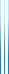# PublicationsManuscripts
For $n$ up to 5, we have compiled a list of all NPN-equivalence classes of Boolean functions on $n$ variables. Each such function is given as a hexadecimal integer in which bit $i$ is the value at the $i$th input.